Updated by mercedesamca on Apr 23, 2018
REPORT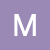mercedesamca
Owner
11 items   3 followers   0 votes   605 views

# Digital Math Resources with Premade Quizizz

These resources come with a premade quiz in Quizizz which helps you get math data without grading a single problem!

1

## Multiplication Facts, Strategies, and Games for the Google Classroom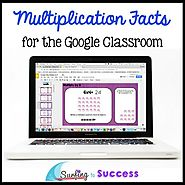Improve memorization and help students multiply fluently with this digital Google Slides Resource by focusing on repeated addition and other multiplication strategies. Fun, self paced, differentiated multiplication practice motivates students to pass each assessment.

2

## Division Facts, Strategies, and Games for the Google Classroom | TpT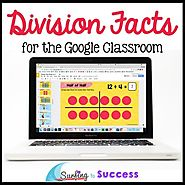Improve memorization and help students divide fluently with this digital Google Slides Resource by focusing on unknown factor and other division strategies. Division strategies include using arrays, creating equal groups, fact families, skip counting, using multiplication to divide, strip diagrams, half, half of half for

3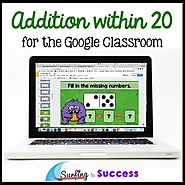Are your students fluent in addition facts? Students will add within 5, add within 10, make ten, and add within 20 using several strategies in Google Slides. Animated directions allow students to work independently on Part Part Whole, Number Lines, Turn Around Facts, Ten Frames, Twenty Frames and more.

4

## Subtract within 20: Subtraction Facts and Strategies for the Google ClassroomAre your students fluent in subtraction facts? Students will subtract within 5, subtract within 10, and subtract within 20 using several strategies in Google Slides. Animated directions allow students to work independently on Part Part Whole, Number

5

## Order of Operations PEMDAS Interactive Math for the Google Classroom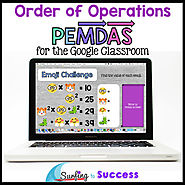PEMDAS is a common acronym for the Order of Operations. This digital resource for the Google classroom, is scaffolded to encourage independent learning. Students practice progressively more challenging order of operations problems including Addition & Subtraction, Multiplication & Division, parentheses and exponents.

6

## Compare Fractions: Interactive Math for the Google Classroom | TpT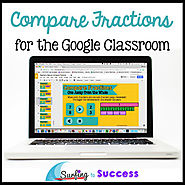5 strategies for comparing fractions are covered in this digital Google Slides resource. Compare Fractions Using Models Compare Fractions with a Common Denominator
Compare Fractions with a Common Numerator
Compare Fractions using Half as a Benchmark
Compare Fractions that are One Away from the Whole

7

## Equivalent Fractions: Interactive Math for the Digital Classroom5 strategies for finding equivalent fractions are covered in this digital Google Slides resource. After reviewing fraction vocabulary, students will recognize and generate equivalent fractions using fraction bars, fraction tiles, fraction number lines, and multiplication.

8

## Multiply Multi-Digit Numbers Area Model & Partial Product for Google Classroom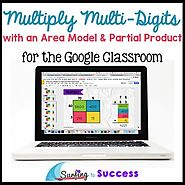This digital interactive math resource for your Google classroom helps your students learn to multiply multi-digit numbers using the area model (also known as box method) and Partial Product Algorithm. There are four levels: Two Digit by One Digit Multiplication, Three Digit by One Digit Multiplication, Two Digit by Two Digit Multiplication, and Three Digit by Two Digit Multiplication.

9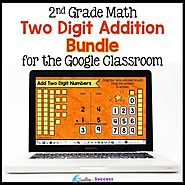Are your 2nd graders learning to do 2 digit addition with regrouping? This digital resource teaches multiple strategies for two digit addition including adding using a number line, add two digit numbers by decomposing numbers (also known as add with expanded form), addition with place value manipulatives, and adding two digit numbers using the standard algorithm.

10

## Two Digit Subtraction with Regrouping BUNDLE for the Google Classroom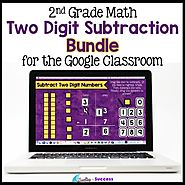Are your 2nd graders learning to do 2 digit subtraction with regrouping? This digital resource teaches multiple strategies for two digit subtraction including subtraction using a number line, subtract two digit numbers by decomposing numbers (also known as subtract with expanded form), subtraction with place value manipulatives, and subtracting two digit numbers using the standard algorithm.

11

## Long Division for the Google Classroom by Mercedes Hutchens | TpT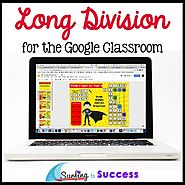Looking for long division practice for your 4th grade and 5th grade students? Help your student learn to divide using the standard algorithm. Students divide whole numbers with 6 levels starting with 3 digit dividends and a one digit divisor. Divide 3 Digits by 1 with remainders and without | Divide 4 Digits by 1 with remainders and without | Divide Four